# Fraction calculator

The calculator performs basic and advanced operations with fractions, expressions with fractions combined with integers, decimals, and mixed numbers. It also shows detailed step-by-step information about the fraction calculation procedure. Solve problems with two, three, or more fractions and numbers in one expression.

## Result:

### 1/3 + 2/5 = 11/15 ≅ 0.7333333

Spelled result in words is eleven fifteenths.

### How do you solve fractions step by step?

1. Add: 1/3 + 2/5 = 1 · 5/3 · 5 + 2 · 3/5 · 3 = 5/15 + 6/15 = 5 + 6/15 = 11/15
For adding, subtracting, and comparing fractions, it is suitable to adjust both fractions to a common (equal, identical) denominator. The common denominator you can calculate as the least common multiple of both denominators - LCM(3, 5) = 15. In practice, it is enough to find the common denominator (not necessarily the lowest) by multiplying the denominators: 3 × 5 = 15. In the following intermediate step, it cannot further simplify the fraction result by canceling.
In other words - one third plus two fifths = eleven fifteenths.

#### Rules for expressions with fractions:

Fractions - simply use a forward slash between the numerator and denominator, i.e., for five-hundredths, enter 5/100. If you are using mixed numbers, be sure to leave a single space between the whole and fraction part.
The slash separates the numerator (number above a fraction line) and denominator (number below).

Mixed numerals (mixed fractions or mixed numbers) write as integer separated by one space and fraction i.e., 1 2/3 (having the same sign). An example of a negative mixed fraction: -5 1/2.
Because slash is both signs for fraction line and division, we recommended use colon (:) as the operator of division fractions i.e., 1/2 : 3.

Decimals (decimal numbers) enter with a decimal point . and they are automatically converted to fractions - i.e. 1.45.

The colon : and slash / is the symbol of division. Can be used to divide mixed numbers 1 2/3 : 4 3/8 or can be used for write complex fractions i.e. 1/2 : 1/3.
An asterisk * or × is the symbol for multiplication.
Plus + is addition, minus sign - is subtraction and ()[] is mathematical parentheses.
The exponentiation/power symbol is ^ - for example: (7/8-4/5)^2 = (7/8-4/5)2

#### Examples:

subtracting fractions: 2/3 - 1/2
multiplying fractions: 7/8 * 3/9
dividing Fractions: 1/2 : 3/4
exponentiation of fraction: 3/5^3
fractional exponents: 16 ^ 1/2
adding fractions and mixed numbers: 8/5 + 6 2/7
dividing integer and fraction: 5 ÷ 1/2
complex fractions: 5/8 : 2 2/3
decimal to fraction: 0.625
Fraction to Decimal: 1/4
Fraction to Percent: 1/8 %
comparing fractions: 1/4 2/3
multiplying a fraction by a whole number: 6 * 3/4
square root of a fraction: sqrt(1/16)
reducing or simplifying the fraction (simplification) - dividing the numerator and denominator of a fraction by the same non-zero number - equivalent fraction: 4/22
expression with brackets: 1/3 * (1/2 - 3 3/8)
compound fraction: 3/4 of 5/7
fractions multiple: 2/3 of 3/5
divide to find the quotient: 3/5 ÷ 2/3

The calculator follows well-known rules for order of operations. The most common mnemonics for remembering this order of operations are:
PEMDAS - Parentheses, Exponents, Multiplication, Division, Addition, Subtraction.
BEDMAS - Brackets, Exponents, Division, Multiplication, Addition, Subtraction
BODMAS - Brackets, Of or Order, Division, Multiplication, Addition, Subtraction.
GEMDAS - Grouping Symbols - brackets (){}, Exponents, Multiplication, Division, Addition, Subtraction.
Be careful, always do multiplication and division before addition and subtraction. Some operators (+ and -) and (* and /) has the same priority and then must evaluate from left to right.

## Fractions in word problems:

• Mixed to improperChange the given mixed numbers to improper fraction: five-and-four-over-nine (5 4/9)
• Simple equation 5Solve equation with fractions: X × 3/8 = 1/2
• Equation 20In given equation: 8/9-4/5=2/9+x, find x
• Spending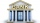Peter spends 1/5 of his earnings on his rent, and he saves 2/7. What fraction of his earnings is left?
• Two cakesTwo cakes were each cut into 8 slices. Maria ate 1/8 of the chocolate cake and 1 slice of carrot cake. Julia ate 1/2 of the carrot cake. Mark ate 1 slice of each. Thomas ate 3 slices of chocolate cake. How many slices were left?
• EquationSolve equation and check the result: 1.4x - 3/2 + x - 9,8 = x + 0,4/3 - 7 + 1,6/6
• Ratio 11Simplify this ratio 10 : 1/4
• How many 3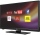How many hours the Andersons watched TV in all Wednesday 3/1 hr Thursdays 2/3 hr Friday 4/5 hr Saturday 3/4 hr
• Seeds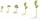Of the 72 wet seeds, 7/8 germinated. How many seeds germinate?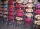Cris had 15000 . He spent 1/3 of his money on a table and the remaining on 5 similar chairs that cost the same. How much did one chair cost?Farmer Peter paints 12 chicken coop. He started painting this day morning. Now he only has 1/4 of the chicken coop left to paint this afternoon. How many chicken coops did farmer Peter paint this morning?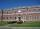At the college are at 45 rooms 158 students. Some rooms are triple and some quad. How many triple and quadruple rooms there is, if it is all fully occupied?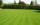Mr. Happy planted 36.6 meters square gardens grass; It's a third of the garden more than half of the garden. What is square area of this garden?